Refereed Conference and Journal Papers of Dr Harvey Cohen in Physics and Geophysics

-->
PhD

ANU
Harvey Alan Cohen, Dispersion Theoretic Approach to Graph Theories of Charged Particles of Spin 0, 1/2, 1 , Instute of Advanced Study, Australian National University, May 1965.
Chapter 2     A New Approach to Calculations in the Quantum Field Theory of Charged particles    opens as follows:
The calculations we perform in subsequent chapters comprise the first exposition of quantum electrodynamics that does not involve the concept of renormalisation and yet does not reject graph theory. Two principles guide us:
Principle A asserts the validity of the dispersion relations appropriate to the quantities to be calculated, some of these DR being well known, others postulated by us for the first time. This principle is used to help us ascertain the graph elements for new theories as well as providing our basic computational device.
Principle B asserts the correctness of the well-known graph elements for Dirac spin 1/2, scalar spin zero, and 5x5 β spin zero, and prescribes that as much of Lagrangian formalism and Dyson-Wick procedures as seem substantive be utilised to determine the graph elements for previously unconsidered theories.

Published Papers on Theoretical and Mathematical Physics

H. A. Cohen, Covariant Wave Equations for Charged Particles of Higher Spin in an Arbitrary Gravitational Field, Il Nuovo Cimento, Serie X, Vol 52A, 1967 pp 1242-1253
Summary It is known that special difficulties are encountered in devising a wave equation to describe higher spin S≥3/2 particles in interaction with the electromagnetic field, and Buchdahl has shown that like difficulties arise when the gravitational field is introduced. We show that just as a consistent electromagnetic interaction can be introduced, so wave equations describing charged particles of spin S = 3/2 and of spin S = 2 in a universe endowed with a Riemannian metric can be devised. The paper incorporates an account of Dirac γ matrices in general relativity.
RIASSUNTO (*)
� noto che sorgono pa ri ieolari difficolt� quando si vuole impostare un'equazione d'onda che descriva particelle di spin superiore o uguale a interagenti con un campo elettromagnetico, e Buchdahl ha mostrato come simili difficolt� sorgano anche quando si introduce un campo gravitazionale. In questo articolo si fa vedere che come si pu� introdurre una interazione elettromagnetica coerente, cos� si possano trovare equazioni d'onda descriventi particelle cariche di spin S 2 o S= 2 in un universo dotato di metrica Riemanniana. Nell'articolo si includono considerazioni sulle matrici y di Dirac in relativit� generale.     (*) Traduzione a cura della Redazione.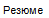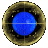The most recent citation of this 1967 publication is this paper on the arXiv preprint registry: [General Relativity and Quantum Cosmology] submitted Wed, 2 Aug 2017. Harold Erbin, Vincent Lahoche, Universality of tunnelling particles in Hawking radiation https://arxiv.org/pdf/1708.00661.pdf [gr-qc]

H. A. Cohen* and J. Smith**, The C-Conserving Decay Modes η → π0e+e- and η → π0μ+μ-
* Mathematical Physics, University of Adelaide
** Institute for Theoretical Physics, State University of New York at Stony Brook
The C-conserving decay rate for η → π0μ+μ- is of second order in the fine structure constant. A knowlege of the matrix elements η →γγ and γγ → e+e- is sufficient to find the imaginary part of the matrix element η → π0μ+μ- from unitarity. The real part of the matrix element can be found from dispersion relations. Recent theoretical estimates (1) of the decay rate for η → π0e+e- have assumed an interaction ηπ0FμνFμν for the ηπ0γγ amplitude.(2) However, this interaction leads to the result that the decay rate into one π0 is proportional to the square of the mass of the leptons. The rate is therefore much larger for muons(3) than for electrons, whereas the contrary conclusion is reached using the simple phase space argument. (which supposes a constant matrix element). One way to modify this result is to note that the C-conserving decay amplitude is decomposible into two invariant amplitudes: the first corresponding precisely to the S-wave interaction given above and the second to the P-wave interaction included in ηπ0PαPβFαμFβμ where P is the η four momentum. In this paper we adopt the second interaction leading to a calculation that is parallel to the calculation(3) by the second author based on the first interaction. We calculate both the η → π0e+e- and η → π0μ+μ- decay rates

H. A. Cohen, Vacuum polarization in the Lee and Yang theory of charged spin one , Il Nuovo Cimento A Series 10 21 Luglio 1968, Volume 56, Issue 2, pp 467-478 Summary: The radiative correction, to ordere 2, to the photon propagator in the Lee and Yang theory of charged spin-one bosons, is calculated in a dispersion-theoretic manner. Some general aspects of the ξ-limiting formalism are discussed. A useful matrix treatment of Lorentz tensors is presented in an Appendix.
Riassunto Calcoliamo, servendoci della teoria della dispersione, la correzione radiativa, di ordinee 2, al propagatore fotonico nella teoria di Lee ed Yang dei bosoni di spin uno carichi. Si discutono alcuni aspetti generali del formalismo ξ-limitante. In un appendice si presenta un utile trattamento matriciale dei tensori di Lorentz.

HINDSIGHT: The Dyson-Wick formalism that produced a manageable theory of elementary charged particles of spin 1/2 and zero spin simply failed to handle spin one QED. Lee and Yang attempted to develop a quantum electrodynamics of charged spin one by introducing a ξ-limiting formalism. Although no actual values were determined for this most speculative spin-1 QED, Lee and Yang proclaimed that their novel theory worked. In this paper I showed that using the Cutkosky approach to finding the (finite) discontinuities across the branch cuts in the complex momentum plane for substitution in the relevant dispersion relation. Contrary to Lee and Yang's claims of adequacy -- these integrals -- unlike those for spin zero and spin 1/2, simply diverged and the ξ-limiting process did not remove the infinities.

A.J. Bracken and H. A. Cohen, Five Classes of Transformations of Dirac Spinors � The Free-Particle Dirac Equation Is Brought to �p0-, �p1-, �p2-, �p3- and �m-Linear� Forms �, Progress of Theoretical Physics, Vol 26, No 1, 1969, pp 816-831.
Abstract: The free-particle Dirac equation has two remarkable features: (1) It is linear in all four components of the energy-momentum pμ, and also in the mass m. (2) For its solutions there are five distinct simple modes of the invariant scalar product in the momentum representation. In this paper, a theorem presented by Case is generalized and used to obtain five classes of transformations of the Dirac equation. Every transformation in a given class has two properties characteristic of the class: (1) The linearity in a corresponding one of the five quantities p�, m is maintained in the transformed equation. (In this way ``p_0-, ``p_1-, ``p_2-, ``p_3- and ``m-linear'' forms of the Dirac equation are obtained.) (2) A corresponding mode of the invariant scalar product is presented. Thus all five classes consist of canonical transformations. Included amongst the ``p_0-linear'' forms are the Foldy-Wouthuysen-Tani equation, and the one commonly attributed to Cini and Touschek, together with equations appropriate to limiting situations other than the non-relativistic and extreme relativistic ones. The ``canonical'' form proposed by Chakrabarti is of the ``m-linear'' type. Belonging to all three of the ``p1-, ``p2- and ``p3-linear'' categories is a ``p-linear'' form of significance for large |p|.

A.J. Bracken and H. A. Cohen, On Canonical SO(4,1) Transformations of the Dirac Equation, Journal of Mathematical Physics, Vol 10, No 11, Nov. 1969, pp 2024. Abstract: Certain matrix transformations of the free-particle Dirac equation are described as momentum dependemt SO(4,1) transformations. Such of these belonging to any one of five subgroups G(α)(α=0,1,2,3,4,5) are canonical, preserving the Lorentz-invariant product in a corresponding one of five modes of expression. The Dirac equation itself is linear in all five components pα[pμ(μ=0,1,2,3) is the four-momentum operator, p4=m ] of the "five-vector" p~, and a transformation in G(β) has the additional property that the component pβ appears linearly also in the transformed equation. The Mendlowitz and the Foldy-Wouthuysen-Tani accordingly are in G(0), the SO(4) subgroup; and that proposed by Chakrabarti is in G(4), the SO(3,1) subgroup associated with homogeneous Lorentz transformations. For any p~` obtained from p~ by a momentum-dependent Lorentz transformation, there is a corresponding transform of the Dirac equation. Where pα appears in the transformed equation, p appears in the transformed equation. The ambiguities which arise in the specification of the transform leading to a given such equation are associated with the existence of a "little group" for any such p~`.

1971

H. A. Cohen, On the Derivation of the Pryce-Foldy-Wouthuysen transformation, American Journal of Physics, Vol 39, April 1971, pp 409-412.
Abstract: A simple and instructive derivation of the Pryce-Foldy-Wouthuysen transformation is given in terms of rotations in two dimensions.

1972

H. A. Cohen, On Time Non-Special Quantum Mechanics, Acta Physica Austrica, Suppl. IX, 1972 pp 851-855.
Abstract: A reformulation of quantum mechanics is described in which the z co-ordinate is described in which the z co-ordinate has the special role with regard to the three other space-time co-ordinates that the time t has in the usual formulation.

1973.1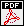E.F. Carter and H. A. Cohen, The Classical Problem of Charge and Pole, American Journal of Physics, Vol 41, Issue 8, Aug 1973, pp 944-950.
Abstract: In this paper the classical dynamics of interacting electrically charged particles where one or both possess magnetic charge is reviewed. The equations of motion are obtained, and the vector constants of motion are derived. A consistent canonical formalism is developed while related aspects such as scale invariance and the geometry of the motion are also discussed.

1973.2H. A. Cohen, On the Dirac Monopole Potential, Prog. Theor. Phys. Vol. 50 No. 2 (1973) pp. 691-696
Abstract: The Grönblom-Jordan picture of the Dirac magnetic monopole is reviewed. The role of the string in a single-valued vector potential describing a magnetic monopole is demonstrated.        First Page Only

1974

H. A. Cohen and M.Z. Shaharir The Action Principle in Quantum Mechanics, International Journal of Theoretical Physics, Vol. 11 No. 5 (1974) pp. 289-303
Abstract: The Euler-Lagrange equation derived from Schwinger's action principle (1951) has been shown by Kianget al. (1969) and Linet al. (1970) to lead to inconsistencies for quadratic lagrangians of the form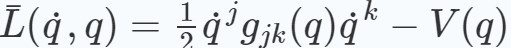except in the Euclidean case gjkjk . This inadequacy is linked to Schwinger's specification that the variations of operators be c-numbers. We reformulate the action principle by introducing the concept of ‘proper’ Gauteaux variation of operators to find the most general class of admissible variation consistent with the postulated quantisation rules. This new action principle, applied to the LagrangianL, yields a quantum Euler equation consistent with the Hamilton-Heisenberg equations.

1974H. A. Cohen, Is There a Quantization Condition for the Classical Problem of Charge and Pole, Foundations of Physics, Vol. 4 No. 1 (1974) pp. 115-120
Abstract: In elementary derivations of the quantization of azimuthal angular momentum the eigenfunction is determined to be exp(imφ), which is "oversensitive" to the rotation φ → φ +2π, unless m is an integer. In a recent paper Kerner examined the classical system of charge and magnetic pole and expressed Π, a vector constant of motion for the system, in terms of a physical angle ψ, to deduce a remarkable paradox. Kerner pointed out that Π(ψ) is "oversensitive" to ψ → ψ +2π, unless a certain charge quantization condition is met. Our explicandum of this paradox highlights the distinction between coordinates in classical and quantum physics. It is shown why the single-valuedness requirement on Π(ψ) is devoid of physical significance. We are finally led to examine the classical analog of the quantum mechanical argument that demonstrates the quantization of magnetic charge, to show that there is "no hope" of a classical quantization condition.

 Published Papers on Geophysics and Remote Observation via Satellite . 1997.1 E.A. Essex, P. Webb, I. Horvath. and H.A. Cohen, The Use of Microsatellites in Monitoring the Ionosphere/Plasmasphere in Proc. COSPAR Colloquium on Microsatellites as Research Tools, Ed. F.-B Hsiao, at National Cheng Hung Uniiversity Tainan, Taiwan, 15-17 December, 1997 COSPAR Colloquia Series 10, pp276-279

 Writings on Qualitative PhysicsH.A. Cohen, The Art of Snaring Dragons, M.I.T. Artificial Intelligence Laboratory Memo Number 338, May 1975. Dragons are formidable problems. challenging, and yet solvable using little or even no algebra or calculus. Dragons are also distinguished (unlike other physics problems seen by the tyro as "difficult") by the fact that both the tyro - and the experienced physicist, are confident with their - so-different - predictions. Thus such Dragons are akin to the Conservation Puzzles of Piaget, where both the four-year old pre-conserver, and the six year conserver are equally confident with their (differing!) conclusions. In this paper, written when the writer was part of the LOGO Group at the MIT Artificial Intelligence Laboratory, an explanation is given in terms of the Frames concept of Marvin Minsky. H.A. Cohen, with illustrations by Jeni Rawson, A Dragon Hunter"s Box, Hanging Lake Books, Melbourne, 1974. { A componendium of qualitative problems in dynamics }H.A. Cohen, Mathematical Dragon Hunting on the La Trobe Campus, Australian Vice-Chancellor"s Committee (AVCC) Educational Newsletter, 3/73, January 1974. To stimulate the intellectual growth of students a new style of student exercise, the Dragon, has been devised and utilised in a course in applied mathematics at La Trobe University.

 DIRECTORYPublications Since 89 By Year This is a collection of papers in image engineering and visual information systems, including image processing generally including numberplate recognition and speed-up issues, image restoration, (IFS and) fractal image coding, issues in image retrieval using thumbnails, and parallel processing techniques, together with some papers devoted to the design of image codecs, to aspects of satellite systems and to the education of engineers.Publications Prior to 89 By Topic excluding Physics Papers These papers are grouped by topic. Topic titles include qualitative physics, mathematics education, OZNAKI - a pioneering educational robotics project, the La Trobe Talking Communicator ( developed for severely disable non-speech students ).Papers on Physics and Geophysics This directory of papers in theoretical particle physics and quantum electrodynamics includes the abstracts for each paper.Primers on VIS and Image Engineering VIS = Visual Information Systems.Topics: Edges. Interesting points. Image restoration. Image access via thumbnails. Texture analysis, identification, segmentation. Fuzzy image processing. Using fractals to encode images. The perceptron images.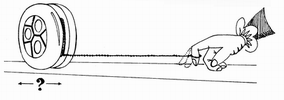Dragons are formidable problems in qualitative physics, challenging, and yet solvable using little or even no algebra or calculus. e.g. Which direction does the yo-yo roll? What if the table were greased?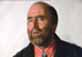Personal Home Page of Dr Harvey A. Cohen of Melbourne Australia